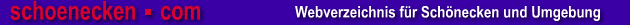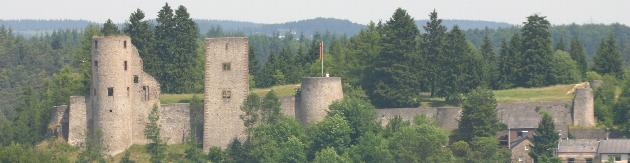Amerika-Auswanderer aus dem Amt Schönecken im 19. und 20. Jahrhundert
- Destination Codes / Zielcodes für USA/Kanada und Bermudas -
 173 = Gloversville 263 = G. Rapids 357 = Hartford 578 = North Hampton 637 = Fall River 1200 = Florida 1432 = Kansas City 1756 = Latrobe, PA 1933 = Kansas 1987 = Houston, TX 2316 = Chicago, Ill 2316 = Illinois 2333 = Wardell, Idaho 2399 = Iron Belt Wisc. 2401 = Council Bluffs, IA 2401 = Iowa 2412 = Indiana 2577 = Boston 2577 = Washington, DC 2764 = California 2880 = Canada 2985 = Brooklyn, NY 2993 = Buffalo 3455 = Bermuda 3465 = Bethlehem 3561 = Bakersfield, CA 3801 = Denver 3917 = Dallas 3595 = Baltimore 3948 = Pittsburgh, PA 3948 = Dakota 4135 = Duluth 4146 = Plymouth, Mass 4325 = Charleston, SC 4434 = Chicago, Ill 4434 = Rochester, NY 4604 = Connecticut 4636 = Buffalo 4779 = Coal City, IL 4810 = Cleveland, Ohio 4819 = Detroit 4950 = Scranton, PA 5023 = Sharpsburg 5098 = San Jose, Cal 5115 = San Francisco 5231 = Springfield 5236 = Spring Valley, Ill 5348 = Stockton, Calif 5558 = Providence, RI 5558 = Rhode Island 5662 = Redding, PA 5930 = St. Louis 5963 = St. Paul 6207 = Wheeling 6255 = West Virginia 6281 = Washington, DC 6281 = New York 6462 = Worcester, MA 6767 = Texas 6678 = Richmond, VA 6678 = Wisconsin 6772 = Tennessee 6825 = N. Tarrytown, NY 6838 = Toronto 6851 = Toluca Illinois 6872 = Topeka, KS 6981 = Syracuse 7039 = Vermont 7173 = Virginia 7244 = Trenton, NJ 7253 = Troy 7327 = Utah 7328 = Hartford, Conn. 7328 = United States 7354 = Montana 7359 = Monongahela, PA 7440 = Morristown, NJ 7535 = Milwood, PA 7554 = Michigan 7574 = Minnesota 7585 = Minneapolis 7598 = Missouri 7611 = Mississippi 7637 = Milwaukee 7670 =Batavia, NY 7670 = Batavia, NY 7670 = New Jersey 7670 = Worcester, MA 7686 = New Haven, CT 7689 = Nevada 7777 = New Orleans 7778 = New York 7788 = Albany 7788 = Jersey 7788 = New York City 7788 = Providence, RI 7788 = Cincinnati 7789 = Coal City, IL 7802 = Jamestown, NY 7802 = Newark 7913 = Nebraska 8035 = Maine 8177 = Louisville 8181 = Long Island 8405 = Meriden 8488 = Manitoba 8527 = Dedham, MA 8527 = Worcester, MA 8527 = Cincinnati 8527 = Massachusetts 8555 = Pennsylvania 8581 = Carbondale 8581 = Paterson, NJ 8581 = Philadelphia 8581 = Richmond, VA 8667 = Plymouth, Mass 8706 = Pittsfield 8707 = Pittsburgh, PA 8776 = Paterson, NJ 8855 = Pennsylvania 8914 = Providence, RI 8992 = Racine 8995 = Quincy 9112 = Poughkeepsie 9311 = Illinois 9311 = Ohio 9672 = Oregon 9682 = Ontario 9311 = Irondale, Ohio 9417 = Norway, Mich 9542 = Oneida, NY 9872 = Albany
 nach oben Geschichtshauptseite Auswandererhauptseite Startseite